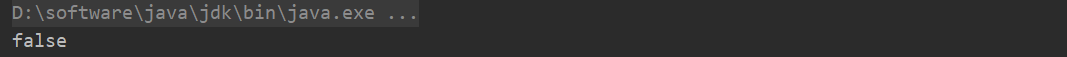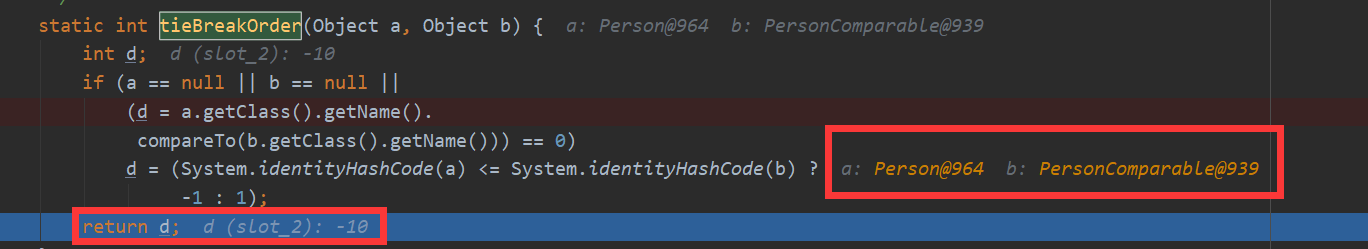# Java入门系列之集合HashMap源码分析

## HashMap原理分析

public class HashMap<K,V> extends AbstractMap<K,V>
implements Map<K,V>, Cloneable, Serializable {

//默认初始化容量
static final int DEFAULT_INITIAL_CAPACITY = 1 << 4;

//最大容量
static final int MAXIMUM_CAPACITY = 1 << 30;

//默认负载因子
static final float DEFAULT_LOAD_FACTOR = 0.75f;

//链表转红黑树阈值
static final int TREEIFY_THRESHOLD = 8;

//取消阈值
static final int UNTREEIFY_THRESHOLD = 6;

//最小树容量
static final int MIN_TREEIFY_CAPACITY = 64;

}

### 构造函数分析

    public HashMap() {
}

public HashMap(int initialCapacity) {
}

   public HashMap(int initialCapacity, float loadFactor) {
if (initialCapacity < 0)
throw new IllegalArgumentException("Illegal initial capacity: " +
initialCapacity);
if (initialCapacity > MAXIMUM_CAPACITY)
initialCapacity = MAXIMUM_CAPACITY;
throw new IllegalArgumentException("Illegal load factor: " +
this.threshold = tableSizeFor(initialCapacity);
}

static final int tableSizeFor(int cap) {
int n = cap - 1;
n |= n >>> 1;
n |= n >>> 2;
n |= n >>> 4;
n |= n >>> 8;
n |= n >>> 16;
return (n < 0) ? 1 : (n >= MAXIMUM_CAPACITY) ? MAXIMUM_CAPACITY : n + 1;
}

       0000 0000 0000 0000 0000 0000 0000 0101                                                      cap = 5
0000 0000 0000 0000 0000 0000 0000 0100                                                      n = cap - 1
0000 0000 0000 0000 0000 0000 0000 0010                                                      n >>> 1
0000 0000 0000 0000 0000 0000 0000 0110                                                      n |= n >>> 1
0000 0000 0000 0000 0000 0000 0000 0001                                                      n >>> 2
0000 0000 0000 0000 0000 0000 0000 0111                                                      n |= n >>> 2
0000 0000 0000 0000 0000 0000 0000 0000                                                      n >>> 4
0000 0000 0000 0000 0000 0000 0000 0111                                                      n |= n >>> 4
0000 0000 0000 0000 0000 0000 0000 0000                                                      n >>> 8
0000 0000 0000 0000 0000 0000 0000 0111                                                      n |= n >>> 8
0000 0000 0000 0000 0000 0000 0000 0000                                                      n >>> 16
0000 0000 0000 0000 0000 0000 0000 0111                                                      n |= n >>> 16

## 插入原理分析

public V put(K key, V value) {
return putVal(hash(key), key, value, false, true);
}

static final int hash(Object key) {
int h;
return (key == null) ? 0 : (h = key.hashCode()) ^ (h >>> 16);
}

 final V putVal(int hash, K key, V value, boolean onlyIfAbsent,
boolean evict) {
Node<K,V>[] tab; Node<K,V> p; int n, i;

// 步骤【1】：tab为空扩容
if ((tab = table) == null || (n = tab.length) == 0)
n = (tab = resize()).length;

// 步骤【2】：计算index，并对null做处理
if ((p = tab[i = (n - 1) & hash]) == null)
tab[i] = newNode(hash, key, value, null);
else {
Node<K,V> e; K k;

// 步骤【3】：键存在，直接覆盖值
if (p.hash == hash &&
((k = p.key) == key || (key != null && key.equals(k))))
e = p;

// 步骤【4】：若为红黑树
else if (p instanceof TreeNode)
e = ((TreeNode<K,V>)p).putTreeVal(this, tab, hash, key, value);
else {

// 步骤【5】：若为链表
for (int binCount = 0; ; ++binCount) {
if ((e = p.next) == null) {
p.next = newNode(hash, key, value, null);

//若链表长度大于8则转换为红黑树进行处理
if (binCount >= TREEIFY_THRESHOLD - 1)
treeifyBin(tab, hash);
break;
}
if (e.hash == hash &&
((k = e.key) == key || (key != null && key.equals(k))))
break;
p = e;
}
}
if (e != null) {
V oldValue = e.value;
if (!onlyIfAbsent || oldValue == null)
e.value = value;
afterNodeAccess(e);
return oldValue;
}
}
++modCount;

// 步骤【6】：超过最大容量进行扩容
if (++size > threshold)
resize();
afterNodeInsertion(evict);
return null;
}

if ((p = tab[i = (n - 1) & hash]) == null)
tab[i] = newNode(hash, key, value, null);

0000 0000 0000 0000 0000 0000 0001 1111  hash = 31
0000 0000 0000 0000 0000 0000 0000 1111  n - 1
0000 0000 0000 0000 0000 0000 0000 1111 => 15

0000 0000 0000 0000 0000 0000 0011 1111 hash = 63
0000 0000 0000 0000 0000 0000 0000 1111  n - 1
0000 0000 0000 0000 0000 0000 0000 1111 => 15

0000 0000 0000 0000 0000 0000 0111 1111 hash = 95
0000 0000 0000 0000 0000 0000 0000 1111  n - 1
0000 0000 0000 0000 0000 0000 0000 1111 => 15

final Node<K,V>[] resize() {
Node<K,V>[] oldTab = table;
int oldCap = (oldTab == null) ? 0 : oldTab.length;
int oldThr = threshold;
int newCap, newThr = 0;
if (oldCap > 0) {
if (oldCap >= MAXIMUM_CAPACITY) {
threshold = Integer.MAX_VALUE;
return oldTab;
}
else if ((newCap = oldCap << 1) < MAXIMUM_CAPACITY &&
oldCap >= DEFAULT_INITIAL_CAPACITY)
newThr = oldThr << 1;
}
else if (oldThr > 0)
newCap = oldThr;
else {
newCap = DEFAULT_INITIAL_CAPACITY;
}
if (newThr == 0) {
float ft = (float)newCap * loadFactor;
newThr = (newCap < MAXIMUM_CAPACITY && ft < (float)MAXIMUM_CAPACITY ?
(int)ft : Integer.MAX_VALUE);
}
threshold = newThr;
......
}

int index = (n - 1) & hash;

int index = (hash & 0x7FFFFFFF) % tab.length;

### 重哈希源码分析

  Node<K,V>[] newTab = (Node<K,V>[])new Node[newCap];
table = newTab;
if (oldTab != null) {
for (int j = 0; j < oldCap; ++j) {
Node<K,V> e;
if ((e = oldTab[j]) != null) {
oldTab[j] = null;
if (e.next == null)
newTab[e.hash & (newCap - 1)] = e;
else if (e instanceof TreeNode)
((TreeNode<K,V>)e).split(this, newTab, j, oldCap);
else { // preserve order
Node<K,V> loHead = null, loTail = null;
Node<K,V> hiHead = null, hiTail = null;
Node<K,V> next;
do {
next = e.next;
if ((e.hash & oldCap) == 0) {
if (loTail == null)
else
loTail.next = e;
loTail = e;
}
else {
if (hiTail == null)
else
hiTail.next = e;
hiTail = e;
}
} while ((e = next) != null);
if (loTail != null) {
loTail.next = null;
}
if (hiTail != null) {
hiTail.next = null;
}
}
}
}
}
return newTab;
}

                        Node<K,V> loHead = null, loTail = null;
Node<K,V> hiHead = null, hiTail = null;
Node<K,V> next;
do {
next = e.next;
if ((e.hash & oldCap) == 0) {
if (loTail == null)
else
loTail.next = e;
loTail = e;
}
else {
if (hiTail == null)
else
hiTail.next = e;
hiTail = e;
}
} while ((e = next) != null);
if (loTail != null) {
loTail.next = null;
}
if (hiTail != null) {
hiTail.next = null;
}

static final int hash(21) {
int h;
return (21 == null) ? 0 : (h = 21.hashCode()) ^ (h >>> 16);
}

i = (16 - 1) & 21

### 将值插入红黑树保持树平衡

            // 步骤【4】：若为红黑树
else if (p instanceof TreeNode)
e = ((TreeNode<K,V>)p).putTreeVal(this, tab, hash, key, value);

然后我们看看上述将值放入到红黑树中具体方法实现，如下：

        final TreeNode<K,V> putTreeVal(HashMap<K,V> map, Node<K,V>[] tab,
int h, K k, V v) {
Class<?> kc = null;
boolean searched = false;
TreeNode<K,V> root = (parent != null) ? root() : this;
for (TreeNode<K,V> p = root;;) {
int dir, ph; K pk;
if ((ph = p.hash) > h)
dir = -1;
else if (ph < h)
dir = 1;
else if ((pk = p.key) == k || (k != null && k.equals(pk)))
return p;
else if ((kc == null &&
(kc = comparableClassFor(k)) == null) ||
(dir = compareComparables(kc, k, pk)) == 0) {
if (!searched) {
TreeNode<K,V> q, ch;
searched = true;
if (((ch = p.left) != null &&
(q = ch.find(h, k, kc)) != null) ||
((ch = p.right) != null &&
(q = ch.find(h, k, kc)) != null))
return q;
}
dir = tieBreakOrder(k, pk);
}

TreeNode<K,V> xp = p;
if ((p = (dir <= 0) ? p.left : p.right) == null) {
Node<K,V> xpn = xp.next;
TreeNode<K,V> x = map.newTreeNode(h, k, v, xpn);
if (dir <= 0)
xp.left = x;
else
xp.right = x;
xp.next = x;
x.parent = x.prev = xp;
if (xpn != null)
((TreeNode<K,V>)xpn).prev = x;
moveRootToFront(tab, balanceInsertion(root, x));
return null;
}
}
}

                else if ((kc == null &&
(kc = comparableClassFor(k)) == null) ||
(dir = compareComparables(kc, k, pk)) == 0) {
if (!searched) {
TreeNode<K,V> q, ch;
searched = true;
if (((ch = p.left) != null &&
(q = ch.find(h, k, kc)) != null) ||
((ch = p.right) != null &&
(q = ch.find(h, k, kc)) != null))
return q;
}
dir = tieBreakOrder(k, pk);
}

    public class PersonComparable implements Comparable<PersonComparable> {
int age;

public PersonComparable(int age) {
this.age = age;
}

@Override
public boolean equals(Object obj) {
if (this == obj) {
return true;
}

if (obj instanceof PersonComparable) {
PersonComparable p = (PersonComparable) obj;
return (this.age == p.age);
}

return false;
}

@Override
public int hashCode() {
return 42;
}

@Override
public int compareTo(PersonComparable o) {
return this.age - o.age;
}
}

        HashMap hashMap = new HashMap();

Person p1 = new Person(1);
Person p2 = new Person(2);
Person p3 = new Person(3);
Person p4 = new Person(4);
Person p5 = new Person(5);
Person p6 = new Person(6);
Person p7 = new Person(7);
Person p8 = new Person(8);
Person p9 = new Person(9);
Person p10 = new Person(10);
Person p11 = new Person(11);
Person p12 = new Person(12);
Person p13 = new Person(13);

hashMap.put(p1, "1");
hashMap.put(p2, "2");
hashMap.put(p3, "3");
hashMap.put(p4, "4");
hashMap.put(p5, "5");
hashMap.put(p6, "6");
hashMap.put(p7, "7");
hashMap.put(p8, "8");
hashMap.put(p9, "9");
hashMap.put(p10, "10");
hashMap.put(p11, "11");
hashMap.put(p12, "12");
hashMap.put(p13, "13");

// 若实现Comparable接口返回其具体实现类型，否则返回空
static Class<?> comparableClassFor(Object x) {
if (x instanceof Comparable) {
Class<?> c; Type[] ts, as; Type t; ParameterizedType p;
if ((c = x.getClass()) == String.class) // bypass checks
return c;
if ((ts = c.getGenericInterfaces()) != null) {
for (int i = 0; i < ts.length; ++i) {
if (((t = ts[i]) instanceof ParameterizedType) &&
((p = (ParameterizedType)t).getRawType() ==
Comparable.class) &&
(as = p.getActualTypeArguments()) != null &&
as.length == 1 && as == c) // type arg is c
return c;
}
}
}
return null;
}
   //调用自定义实现Comprable接口比较器，从而确定顺序
static int compareComparables(Class<?> kc, Object k, Object x) {
return (x == null || x.getClass() != kc ? 0 :
((Comparable)k).compareTo(x));
}

dir = tieBreakOrder(k, pk);
       static int tieBreakOrder(Object a, Object b) {
int d;
if (a == null || b == null ||
(d = a.getClass().getName().
compareTo(b.getClass().getName())) == 0)
d = (System.identityHashCode(a) <= System.identityHashCode(b) ?
-1 : 1);
return d;
}

Person person1 = new Person(1);
Person person2 = new Person(1);
System.out.println(System.identityHashCode(person1) == System.identityHashCode(person2));public class Person {
int age;

public Person(int age) {
this.age = age;
}

@Override
public boolean equals(Object obj) {
if (this == obj) {
return true;
}

if (obj instanceof Person) {
Person p = (Person) obj;
return (this.age == p.age);
}

return false;
}

@Override
public int hashCode() {
return 42;
}
}
        HashMap hashMap = new HashMap();

PersonComparable  p1 = new PersonComparable (1);
PersonComparable  p2 = new PersonComparable (2);
PersonComparable  p3 = new PersonComparable (3);
PersonComparable  p4 = new PersonComparable (4);
PersonComparable  p5 = new PersonComparable (5);
PersonComparable  p6 = new PersonComparable (6);

Person p7 = new Person(7);
Person p8 = new Person(8);
Person p9 = new Person(9);
Person p10 = new Person(10);
Person p11 = new Person(11);
Person p12 = new Person(12);
Person p13 = new Person(13);

hashMap.put(p1, "1");
hashMap.put(p2, "2");
hashMap.put(p3, "3");
hashMap.put(p4, "4");
hashMap.put(p5, "5");
hashMap.put(p6, "6");
hashMap.put(p7, "7");
hashMap.put(p8, "8");
hashMap.put(p9, "9");
hashMap.put(p10, "10");
hashMap.put(p11, "11");
hashMap.put(p12, "12");
hashMap.put(p13, "13");## 总结

posted @ 2019-11-30 19:54  Jeffcky  阅读(1079)  评论(7编辑  收藏  举报